# Max Pooling with Approximated Spatial Masking¶

The Approximated Spatial Masking technique, presented in the context of the ReLu function, can also be used to formulate an algorithm for max pooling, which is another non-linear operation used in CNN architectures. As was the case with ReLu, ASM is used to get a more accurate result than using the approximated image directly, however as these notes will show, the error is still quite high. Ultimately this is considered a failure case and is presented only because it is vaugly interesting.

What follows is boilerplate as usual

In :
!pip install tabulate

import numpy as np
import scipy.fftpack
import scipy.signal
from IPython.display import display, HTML
from tabulate import tabulate

%matplotlib inline
import matplotlib.pyplot as plt
import matplotlib.image as mpimg
from matplotlib.colors import LinearSegmentedColormap
from matplotlib import animation, rc

np.warnings.filterwarnings('ignore')

def show_mat(m):
latex = tabulate(m, tablefmt="html",floatfmt=".3f")
display(HTML(data=latex))

def show_image(m, ax=None):
c_img = np.zeros((m.shape, m.shape, 3))

max_gr0 = np.max(m[m > 0])

if len(m[m < 0]) > 0:
min_le0 = np.min(m[m < 0])
else:
min_le0 = 0

c_img[m < 0] = np.array([[0.0, 1.0, 0.0]]) * (m[m < 0] / min_le0).reshape(-1, 1)
c_img[m == 0] = np.array([0.0, 0.0, 1.0])
c_img[m > 0] = np.array([[1.0, 0.0, 0.0]]) * (m[m > 0] / max_gr0).reshape(-1, 1)

if ax is None:
plt.grid(False)
return plt.imshow(c_img)
else:
return ax.imshow(c_img)

Requirement already satisfied: tabulate in /usr/local/lib/python3.6/dist-packages (0.8.2)


## Max Pooling¶

Max Pooling takes a window of pixels in an image $I$ and generates a smaller image $I'$ such that the pixels in $I'$ are the maximum value over the window in $I$. The most common case is $2 \times 2$ max pooling, and without loss of generality, is what is considered here.

In :
def max_pool_2x2(m):
p = np.zeros((m.shape // 2, m.shape // 2))
for i in range(0, m.shape, 2):
for j in range(0, m.shape, 2):
p[i // 2, j // 2] = np.max(m[i:(i+2),j:(j+2)])
return p


Next we make a random $8 \times 8$ image as usual to use as test data

In :
im = np.random.rand(8, 8) * 2 - 1
show_image(im);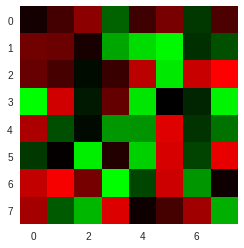and take the $2 \times 2$ max pooling of it, resulting in a $4 \times 4$ image.

In :
spatial_maxpool = max_pool_2x2(im)
show_image(spatial_maxpool);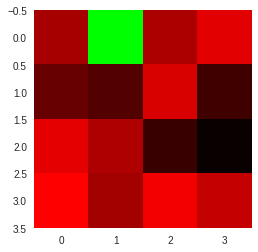## ASM For Max Pooling¶

The ASM method is simple enough. We compute the following mask for an image $I$

$$\text{lmm}_{2, 2}(i, j) = \left\{\begin{array}{lr} 1 & I(i, j) \; \text{is the argmax over the window} \\ 0 & \text{Otherwise} \end{array}\right.$$

we call this the $2 \times 2$ Local Maximum Mask of the image I. This mask is then applied to the image and the result is $2 \times 2$ downsampled by simply adding all the pixels in the $2 \times 2$ windows. Since the non-maximum pixels were zeroed during the mask application, only the local maximum is copied.

Since this is an ASM method, instead of computing the mask from the true image, we use the approximate image by reducing the number of spatial frequencies used for reconstruction.

In :
def normalize(N):
n = np.ones((N, 1))
n[0, 0] = 1 / np.sqrt(2)
return (n @ n.T)

def harmonics(N):
spatial = np.arange(N).reshape((N, 1))
spectral = np.arange(N).reshape((1, N))

spatial = 2 * spatial + 1
spectral = (spectral * np.pi) / (2 * N)

return np.cos(spatial @ spectral)

def dct(im):
N = im.shape

n = normalize(N)
h = harmonics(N)

coeff = (1 / np.sqrt(2 * N)) * n * (h.T @ im @ h)

return coeff

def idct(coeff):
N = coeff.shape

n = normalize(N)
h = harmonics(N)

im = (1 / np.sqrt(2 * N)) * (h @ (n * coeff) @ h.T)

return im


Then implement the approximated local maximum mask, along with a helper function to compute the true mask for error checking.

In :
def almm(dct_im, n_freqs):
appx_im = np.zeros_like(dct_im)
N = dct_im.shape
h = harmonics(N)
n = normalize(N)

for x in range(N):
for y in range(N):
accum = 0
for i in range(8):
for j in range(8):
if i + j <= n_freqs:
accum += n[i, j] * dct_im[i, j] * h[x, i] * h[y, j]

appx_im[x, y] = (1 / np.sqrt(2 * N)) * accum

for i in range(0, appx_im.shape, 2):
for j in range(0, appx_im.shape, 2):
ind = np.unravel_index(np.argmax(appx_im[i:(i+2),j:(j+2)]), (2, 2))

mask[ind + i, ind + j] = 1

def true_lmm(im):

for i in range(0, im.shape, 2):
for j in range(0, im.shape, 2):
ind = np.unravel_index(np.argmax(im[i:(i+2),j:(j+2)]), (2, 2))

mask[ind + i, ind + j] = 1



And then give an example of the mask with 6 spatial frequencies, the same choice that gave a good result for ReLu.

In :
dct_im = dct(im)

plt.figure(figsize=(20, 20))

plt.subplot(1, 3, 1)
plt.title('Original Image')
show_image(im)

plt.subplot(1, 3, 2)

plt.subplot(1, 3, 3)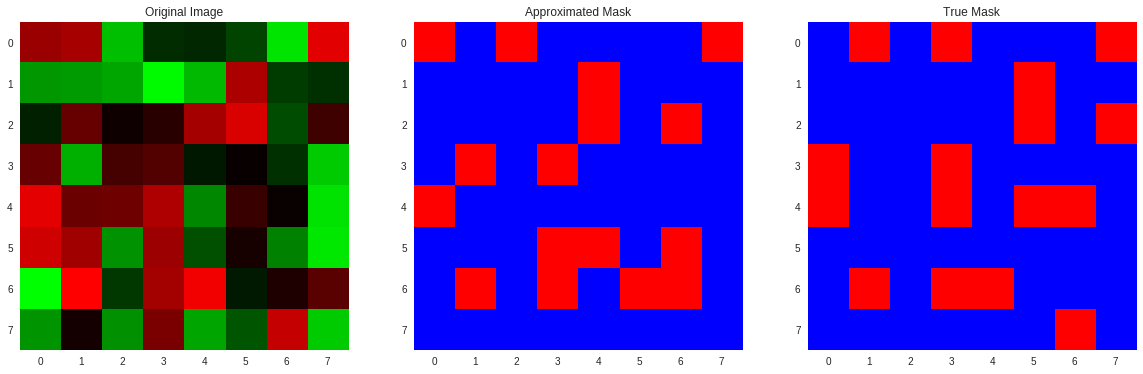Next, a simple function that operates in the spatial domain and computes the max pooling result by applying a local maximum mask, this is the summing method described at the beginning of this section.

In :
def masked_max_pool(im, mask):

p = np.zeros((im.shape // 2, im.shape // 2))
for i in range(0, im.shape, 2):
for j in range(0, im.shape, 2):
p[i // 2, j // 2] = np.sum(masked[i:(i+2),j:(j+2)])
return p



Finally we characterize the error in the same way as the ReLu notes, first with an example

In :
def error(a, b):
return np.sqrt(np.mean((a - b)**2))

plt.figure(figsize=(10, 10))
plt.subplot(1, 2, 1)
plt.title('Approximated Max Pooling')
show_image(appx_mp)

plt.subplot(1, 2, 2)
plt.title('True Max Pooling')
show_image(true_mp)

print('Error: {}'.format(error(appx_mp, true_mp)))

Error: 0.5830967851328782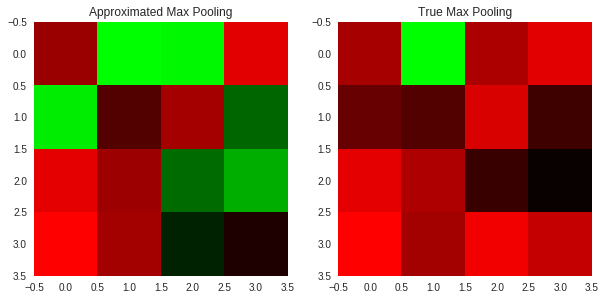It's really bad, lets look at the error as a function of the number of spatial frequencies used in the reconstruction.

In :
error_samples = np.zeros(15)
n_trials = 1000
for i in range(15):
for j in range(n_trials):
im_i = np.random.rand(8, 8)
lmm = true_lmm(im_i)
dct_lmm = almm(dct(im_i), i)

error_samples /= n_trials

plt.figure(figsize=(20, 5))
plt.plot(error_samples)
plt.title('Error vs Number of Spatial Frequencies')
plt.xlabel('# Spatial Frequencies')
plt.ylabel('Error');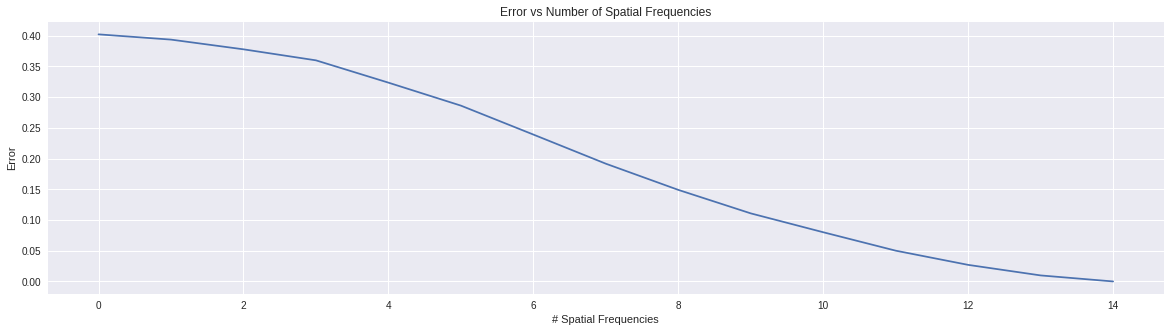The error is pretty high, right up to the full 14 spatial frequencies

## Why Is It So Bad?¶

So the error is quite high and there are two main reasons for it. The first is that the result is a $4 \times 4$ image, so one incorrect pixel throws off the error metric a lot more than in an $8 \times 8$. The next reason is that while ReLu depended on only single pixels signs being correct to produce a correct mask, the max pooling mask requires the relationship between four of the pixels to be preserved. This is a lot harder to ask for than a single pixels sign. Any slight fluctuation in pixel intensity caused by the approximate reconstruction can throw off an entire block. While the Approximated Spatial Mask is a good attempt at a method for computing certain nonlinear functions on compressed images, this certainly shows a serious shortcomming.